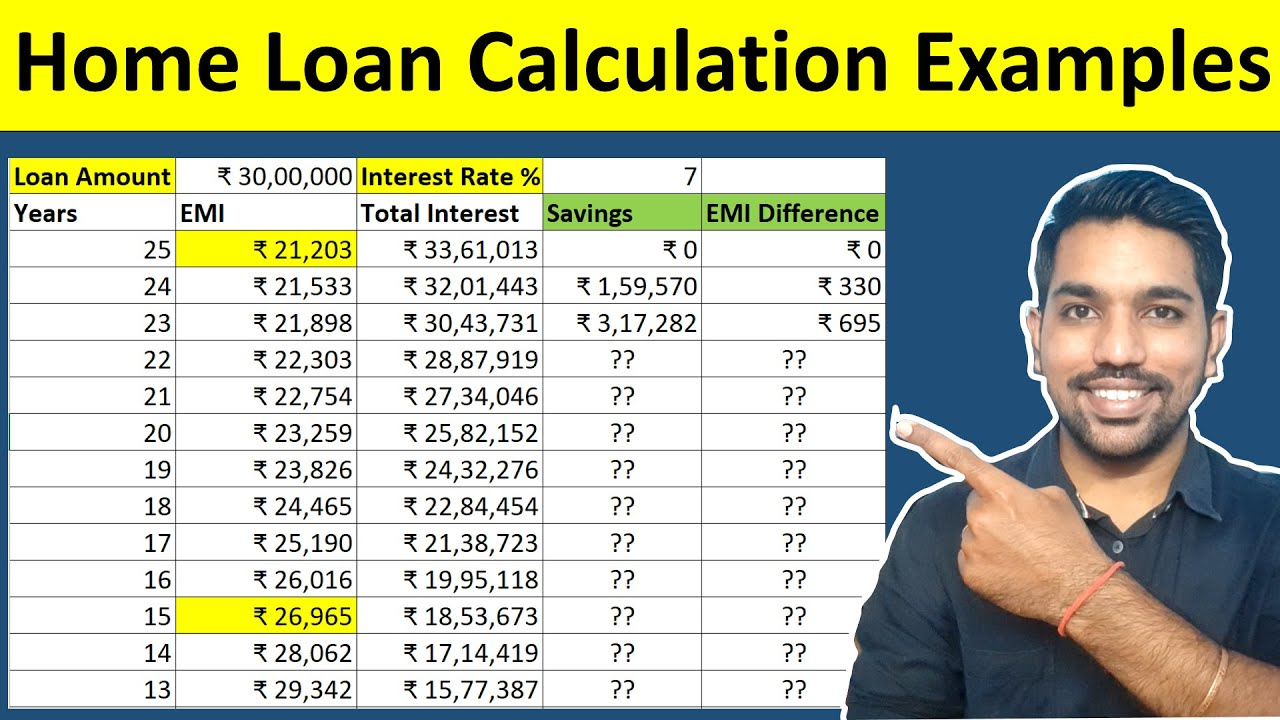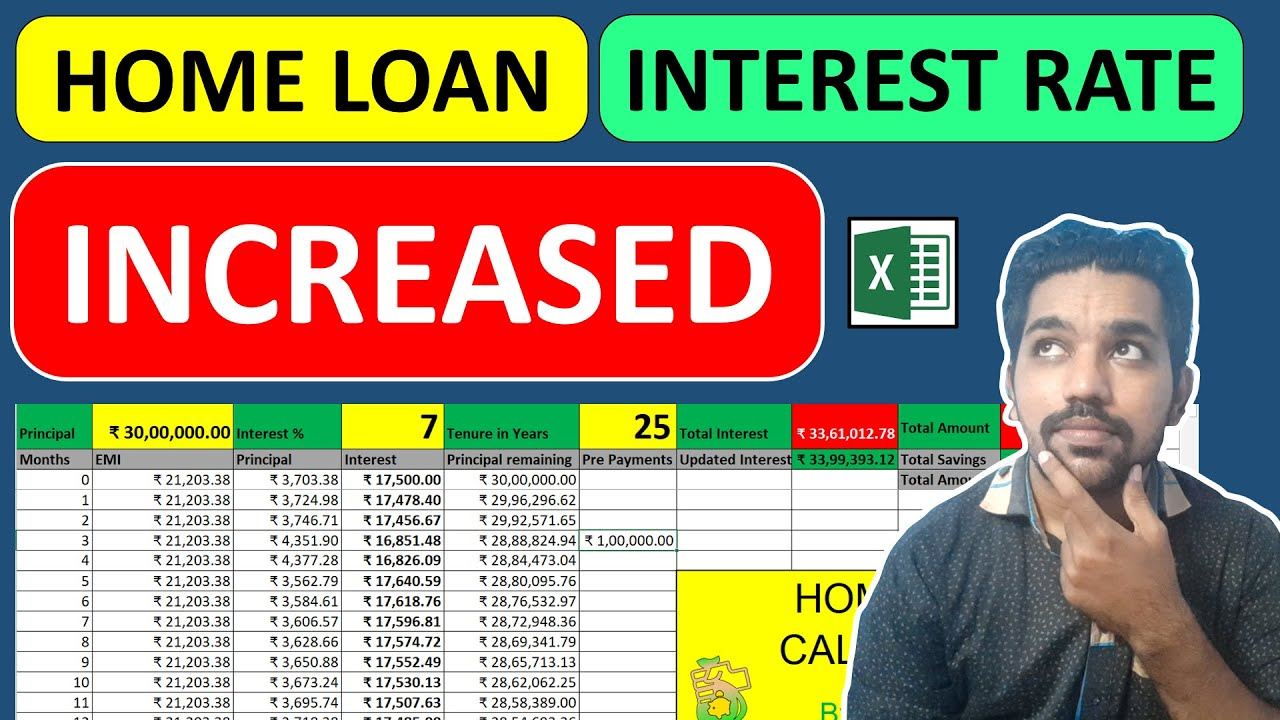# How to Calculate Home Loan EMI [FREE CALCULATOR]One of the most asked questions on my YouTube Channel is how to calculate Home Loan EMI to find out the every month EMI to buy your dream house. Here in this article, I’ll show you the steps to calculate your Home Loan EMI and things you should remember while buying your dream house on Loan or mortgage.

Home Loan EMI calculator helps us to calculate the EMI of the home loan that we will be paying every month. Along with the EMI, the calculator also helps us in finding the total interest we will be paying throughout the loan tenure, with few inputs such as loan principal amount, interest rate and tenure.

Also I’ll provide you the Home Loan Calculator in Excel at the bottom of this article so that you can track the EMI, principal amount, interest amount and principal outstanding amounts every month. You can also make loan prepayments (or part payments) to save home loan interest.

Here’s the video on How to Calculate Home Loan EMI:

## How to Calculate Home Loan EMI Video:As seen in above video, I have explained you the steps to use the Home Loan EMI calculator to calculate the loan EMI! Let us see all the steps.

Steps to use Home Loan EMI Calculator:

• You need to provide 3 inputs here: Loan Amount, Interest rate and Tenure
• Let’s say for example, Loan Amount = Rs. 30 Lacs, Interest Rate = 7% and Tenure = 20 Years (240 months), EMI will be Rs. 23,259, total interest amount will be Rs. 25,82,152

• So in this way you can easily calculate your home loan EMI
• Now let’s you you can afford some more amount to be paid as EMI every month, you can reduce the tenure to 15 years (180 months) and check EMI accordingly:

• As seen above, EMI = Rs. 26,965 and total interest amount is reduced to Rs. 18,53,673. You saved almost Rs. 7,28,479.
That’s one of the ways to save your home loan interest amount. We will see more ways later in this article.

So these were some of the simple steps to calculate your Home Loan EMI. Use the Calculator and you are done!

Let us now see some of the attributes of Home Loan.

## Home Loan Features

• The Home Loan EMI consists of 2 components: Principal Amount and Interest Amount
• Interest Amount is high initially and decreases gradually as you pay EMI every month, which reduces the principal outstanding balance. This reduction in remaining balance helps the interest component of EMI reduce over time
• Principal Amount is low initially and increases gradually as you pay EMI every month
• This doesn’t necessarily mean that Interest amount will be greater than Principal Amount in our Loan EMI
• Interest amount is high during the initial months because we are liable to pay huge amount of loan we have taken from bank or financial institution.
• Whether Interest amount will be more or less than the principal amount of EMI, will depend on the Home Loan Tenure
• Higher the loan tenure, more interest you’ll be paying throughout the loan tenure as seen in above example
• If we reduce the loan tenure, we’ll pay less interest amount overall
• Also, Home Loan prepayments or part payments can help us save our Home Loan Interest

## Factors that Affect Home Loan EMI

Below are some factors that directly affect your Home loan EMI and the total interest amount you’ll be paying throughout loan tenure:

• High interest rate increases the total interest amount you’ll have to pay back. So find banks with low interest rates
• Longer tenure will also increase the total interest amount you have to pay. So lower the tenure so that you can afford the EMI
• Increase in loan Interest rate in case of ARHL (Adjustable Rate home loan or variable rate loan) will either increase loan EMI or tenure on existing loans. So you have to keep an eye on the loan interest rates as well.

Below is the video that explains what happens when home loan interest rate is increased and how you can calculate your updated loan interest amount.

### Home Loan Interest Rate Change Video:Watch more Excel Videos Here

## What is the formula for home loan EMI?

We can calculate Home Loan EMI in multiple ways:

### Mathematical Formula to Calculate Home Loan EMI:

EMI = [P x R x (1+R)N ]/[(1+R)N-1]
where: P = Principal, R = monthly Interest Rate, N = Tenure in months.

Let’s understand this with example. We’ll take same example as taken in our calculator:

P = Rs. 30,00,000
R = 7%
N = 20 Years (240 months)

Putting these figures in the EMI formula:

EMI = [P x R x (1+R)N ]/[(1+R)N-1]
= [30,00,000 * (7 / 12 / 100) * (1 + (7 / 12 / 100)) ^ 240] / [((1 + (7 / 12 / 100)) ^ 240) – 1]
= [30,00,000 * (0.005833) * (1 + (0.005833)) ^ 240] / [((1 + (0.005833)) ^ 240) – 1]
= [30,00,000 * (0.005833) * 4.0387] / [(4.0387) – 1]
= [70,673.89] / [3.0387]
EMI = 23,259

So the EMI using above formula is Rs. 23,259, and this is the same value we got while calculating EMI using our Home Loan EMI Calculator for given example of Principal Amount, Interest rate and Tenure.

Another way to calculate Home Loan EMI is using Excel formula (PMT)

### Excel Formula to Calculate Home Loan EMI:

We can easily use the function pmt to calculate Home loan EMI provided we already know the Principal Amount, Interest rate and Tenure of loan.

So let’s take the same example as above:
P = Rs. 30,00,000
R = 7%
N = 20 Years (240 months)

Putting these numbers in pmt function of Excel, following is how it will look like:

As seen above, pmt function in excel takes 3 parameters:
1. rate = 7 / 12 / 100 – this is the monthly interest rate
2. nper = number of remaining months
3. pv = principal amount of loan taken
4. 4th and 5th parameters are optional so we can pass 0

So using above formula in excel, you can check if the home loan EMI is correct for your loan and also track the same using Excel.

You can also download the home loan Excel calculator that will help you to calculate EMI, make prepayments and save home loan interest and track all the numbers in one excel document.

Enter Correct Email ID to receive Excel
If you opt in above, we use this information to send related contentAnother method to calculate your Home loan EMI is using the online calculator I have made for you.

### Online Calculator to Calculate Home Loan EMI:

I have already explained you how to use this online Home Loan EMI calculator above.

Make sure you provide the required numbers correctly to get monthly EMI and total interest throughout loan tenure accurately.

## What is the EMI for 20 lakhs home loan?

So far we have seen examples of home loan for Rs. 30 Lacs loan. What if you take loan amount of less or more than 30 Lacs?

So in case you opt to take home loan of Rs. 20 Lacs with 7% annual interest rate and tenure = 15 years (180 months), your EMI = Rs. 17,977 and total interest amount = Rs. 12,35,782

More Recommended Books Here

## How is home loan interest calculated?

So we have understood how we can calculate home loan EMI using various methods. Now how do we calculate loan interest amount from this EMI?

Remember, home loan EMI has 2 components: Principal and Interest Amount. So we need to calculate interest amount from EMI and the remaining amount will be principal amount in EMI.

Below is the formula to calculate loan interest amount from loan EMI:

Loan Interest = monthly interest rate * principal Amount remaining / 100

So for our above example of Rs. 30 Lacs loan, interest rate = 7% and tenure = 240 months, EMI was Rs. 23,259. We need to use above formula to calculate loan interest amount from this EMI.

So putting these numbers in formula we get:

Loan Interest = monthly interest rate * principal Amount remaining / 100
= 7% / 12 * Rs. 30,00,000 / 100
= 7% / 12 * Rs. 30,00,000 / 100
Loan Interest = Rs. 17,500

So Rs. 17,500 is the loan interest amount from your first EMI. Remaining amount will be your principal amount = Rs. 5,759 (Rs. 23,259 – Rs. 17,500)

Now in the 2nd month EMI, this principal amount of Rs. 5,759 will be reduced from your principal remaining amount of Rs. 30 Lacs, and interest will be calculated on this updated principal remaining balance.

Updated principal remaining = Rs. 30,00,000 – Rs. 5,759 = Rs. 29,94,241

Now let us again calculate the loan interest for 2nd month EMI:

Loan Interest = monthly interest rate * principal Amount remaining / 100
= 7% / 12 * Rs. 29,94,241 / 100
= 7% / 12 * Rs. 29,94,241 / 100
Loan Interest = Rs. 17,466.40

And Principal Amount will be Rs. 5,792.59 (Rs. 27,259 – Rs. 17,466.40)

So we can observe that loan interest amount is slightly decreased in 2nd month compared to first month, that’s because interest is calculated on reduced principal remaining balance.

On the other hand, principal amount in loan EMI is increased due to decrease in interest portion.

Below is the table showing the principal and interest components for first 5 months for given example:

To get more data on upcoming months, you can use the excel calculator I have provided above.

ALSO READ: Home Loan Tax Benefits

## Conclusion

So to summarize, Home Loan EMI can be easily calculated using 3 methods:

1. Mathematical formula to calculate EMI
2. Excel formula to calculate EMI
3. Online calculator of Home Loan

Apart from calculating home loan EMI, you also need to keep track of the loan interest amounts every month in your EMI and the remaining principal balance of your loan.

It is also important to note that low interest rate, less tenure and loan prepayments (or part payments) are some of the ways to save your home loan interest.

## Home Loan Prepayment VideoWatch more Excel Videos Here

## You can Donate Too!

Found this Helpful? DONATE any amount to see more useful Content. Scan below QR code using any UPI App!

UPI ID: abhilashgupta8149-1@okhdfcbank

Verify that you are “Paying Abhilash Gupta” before making the transaction so that it reaches me. It makes my Day 🙂

Thank you for Donating. Stay Tuned!

## Income Tax Calculator App – FinCalC

For Income Tax Calculation on your mobile device, you can Download my Android App “FinCalC” which I have developed for you to make your income tax calculation easy.

### What you can do with this mobile App?

• Calculate Income Tax for new FY 2023-24 and previous FY 2022-23
• Enter estimated Investments to check income tax with Old and New Tax Regime
• Save income tax details and track regularly
• Know how much to invest more to save income tax
• More calculators including PPF, SIP returns, Savings account interest and lot more

SIP Returns Calculation on Rs. 2,000 SIP

HRA Exemption Calculation

SIP Returns for Next 30 Years

Use Popular Calculators:

I’d love to hear from you if you have any queries about Personal Finance and Money Management.

JOIN Telegram Group and stay updated with latest Personal Finance News and Topics.

Download our Free Android App – FinCalC to Calculate Income Tax and Interest on various small Saving Schemes in India including PPF, NSC, SIP and lot more.